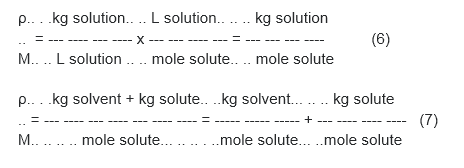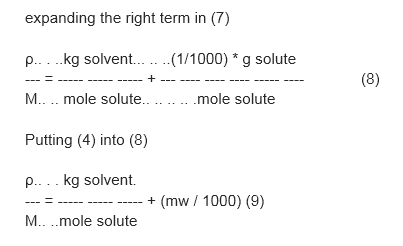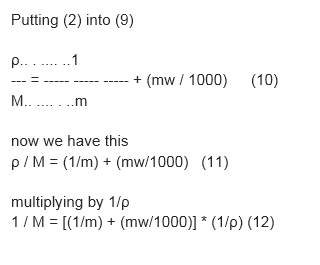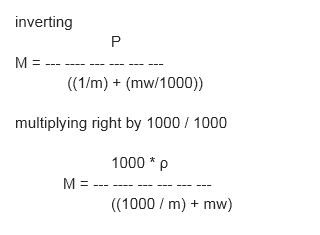Question & Answer
QUESTION

# Derive the relation between molarity and molality

ANSWER

M = molarity = moles solute / Liter solution

m = molality = moles solute / kg solvent

ρ = density = g solution / mL solution = kg solution / L solution

mw = molecular weight (molar mass) of solute in g/mole

"Have a doubt going on your head? Download Vedantu's Instant Doubt Solving App now and get all your doubts cleared by our experts."

Equations:

(1) M = moles solute / L solution

(2)m = moles solute / kg solvent

(3) ρ = kg solution / L solution

(4)mw = g solute / mole solute

(5) kg solution = kg solvent + kg solute

multiplying (3) x 1/(1)